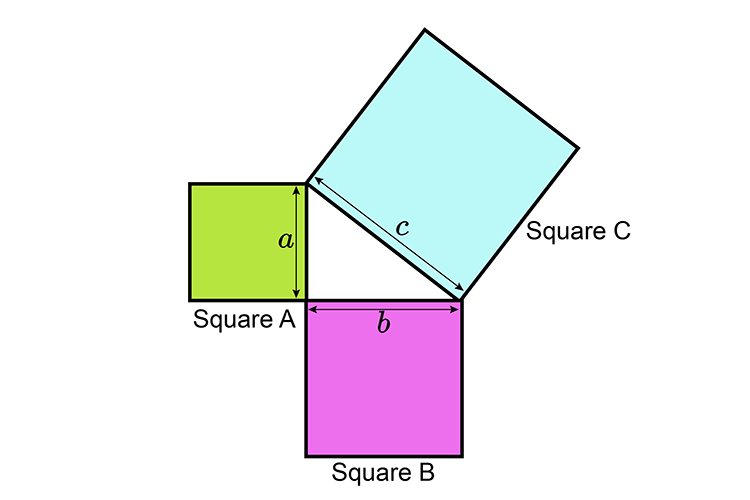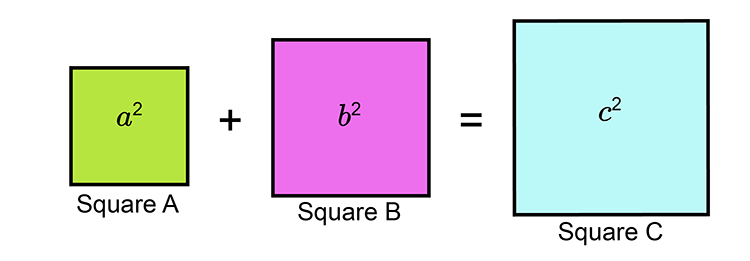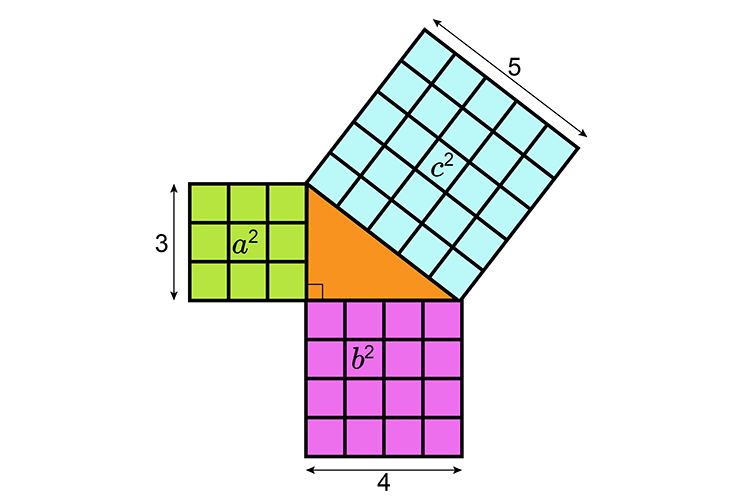# Pythagoras' theorem explanation 2The sum of the squares A+B are exactly the same size as square CThe following diagram shows this more explicitly.a^2+b^2=c^2

Proved by

 3times3 + 4times4 = 5times5 9 + 16 = 25 25 = 25

They call this a three, four, five triangle and it only works with right angled triangles.